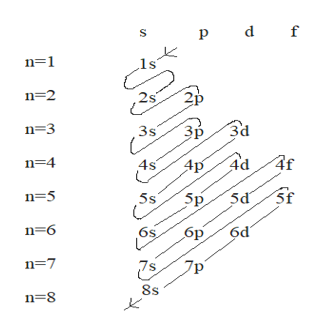# Predict the ground‑state electron configuration of each ion. Use the abbreviated noble gas notation. Cr2+:  Cu2+:   Co3+:

Question
6748 views
Predict the ground‑state electron configuration of each ion. Use the abbreviated noble gas notation.

Cr2+:

Cu2+:

Co3+:

check_circle

Step 1

The electron configuration of an atom is the representation of the arrangement of electrons distributed among the orbital shells and subshells. Commonly, the electron configuration is used to describe the orbitals of an atom in its ground state, but it can also be used to represent an atom that has ionized into a cation or anion by compensating with the loss of or gain of electrons in their subsequent orbitals.

Electrons are filled in the orbitals in a way to minimize the energy of the atom. Therefore, the electrons in an atom fill the principal energy levels in order of increasing energy (the electrons are getting farther from the nucleus). The order of levels filled is:

1s, 2s, 2p, 3s, 3p, 4s, 3d, 4p, 5s, 4d, 5p, 6s, 4f, 5d, 6p, 7s, 5f, 6d and 7p

The maximum number of electrons each orbital can have are: s = 2, p = 6, d = 10, f = 14

A simple way to remember the order is to follow the below pattern :help_outlineImage Transcriptionclosep d f n=1 n 2 n=3 3s Зр 3d n 4 4d 4s 4f Ap n=5 5s Sp 5d n 6 6p 6d n=7 n 8 8s 00 > fullscreen
Step 2

The electronic configuration of Cr2+, using abbreviated noble gas notation can be written as:

The atomic number of chromium is 24. Therefore, total number of electrons in Cr = 24.

Cr (24) : 1s2 2s2 2p6 3s2 3p6 4s2 3d4

Cr (24) : [Ar] 4s2 3d4

Where, [Ar] = electronic configuration of noble gas Argon (18), i.e, 1s2 2s2 2p6 3s2

Now, Cr loses two electrons to become Cr2+, therefore Cr2+ will have two less electrons than Cr, that is, 22 electrons and its electronic configuration will be :

Cr2+ (22) : [Ar] 3d4

Step 3

The electronic configuration of Cu2+, using abbreviated noble gas notation can be written as:

The atomic number of copper is 29. Therefore, total number of electrons in Cu = 29.

Cu (29) : 1s2 2s2 2p6 3s2 3p6 4s1 3d10

Cu (29) : [Ar] 4s1 3d10

Where, [Ar] = electronic configuration of noble gas Argon (18), i.e, 1s2 2s2 2p6 3s2  ...

### Want to see the full answer?

See Solution

#### Want to see this answer and more?

Solutions are written by subject experts who are available 24/7. Questions are typically answered within 1 hour.*

See Solution
*Response times may vary by subject and question.
Tagged in

### General Chemistry